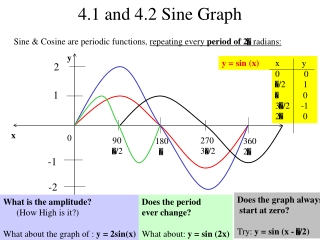DownloadDownload Presentation4.1 and 4.2 Sine Graph

# 4.1 and 4.2 Sine Graph

Download Presentation## 4.1 and 4.2 Sine Graph

- - - - - - - - - - - - - - - - - - - - - - - - - - - E N D - - - - - - - - - - - - - - - - - - - - - - - - - - -
##### Presentation Transcript

1. 90 /2 270 3/2 180  360 2 4.1 and 4.2 Sine Graph Sine & Cosine are periodic functions, repeating every period of 2 radians: y y = sin (x) • x y • 0 0 • /2 1 • 0 3/2 -1 2 0 2 1 x 0 -1 -2 Does the graph always start at zero? Try: y = sin (x - /2) What is the amplitude? (How High is it?) What about the graph of : y = 2sin(x) Does the period ever change? What about: y = sin (2x)

2. 90 /2 270 3/2 180  360 2 y = A sin (Bx – C) amplitude = | A | period = 2 B phase shift = C (right/left) B Equation of a Sine Curve • Graphing Guidelines • Calculate period • 2. Calculate interval • (period / 4) • Calculate phase • shift-‘start-value’ • 4. Create X/Y chart w/ • 5 key points from • ‘start-value’ y = sin (x) y = 2 sin (x) y = sin (2x) y = sin (x - /2) y 2 1 x 0 -1 Does the graph ever shift vertically? – YES y = A sin (Bx – C) + D [D is the vertical shift] What would y = sin (x - /2) + 1 look like? -2

3. 90 /2 270 3/2 180  360 2 Equation of a Cosine Curve y = A cos (Bx – C) amplitude = | A | period = 2 B phase shift = C (right/left) B • x y=cos(x) • 0 1 • /2 0 • -1 3/2 0 2 1 y = cos (x) y = 2 cos (x) y = cos (2x) y = cos (x - /2) y 2 1 x 0 -1 -2

4. 4.3 Equation of a Tangent Function y = A tan (Bx – C) 1. Find & draw two asymptotes Set Bx –C =  and - (solve for x) 2 2 2. Find & plot the x-intercept (Midway between asymptotes) 3. Plot points: x = ¼ from 1st asymptote, y = -A x = ¾ before 2nd asymptote, y = A y = tan(x) 1 0 x   2 - 2 3 2 2

5. Graphing a Cotangent Function y = A cot (Bx – C) 1. Find and draw two asymptotes Set Bx –C = 0 and  (solve for x) 2. Find & plot the x-intercept (Midway between asymptotes) 3. Plot points: x = ¼ from 1st asymptote, y = A x = ¾ before 2nd asymptote, y = -A y = cot(x) 1 0 x   2 - 2 3 2 2

6. 90 /2 270 3/2 180  360 2 4.4 Graphing variations of y=csc(x) csc(x) = 1/sin(x). To graph the cosecant: 1) Draw the corresponding sine graph 2) Draw asymptotes at x-intercepts 3) Draw csc minimum at sin maximum 4) Draw csc maximum at sin minimum y = sin (x) y = csc (x) y 2 1 x 0 -1 -2

7. 90 /2 270 3/2 -/2 180  360 2 450 5/2 Graphing variations of y=sec(x) sec(x) = 1/cos(x). To graph the secant: 1) Draw the corresponding cosine graph 2) Draw asymptotes at x-intercepts 3) Draw sec minimum at cos maximum 4) Draw sec maximum at cos minimum y = cos (x) y = sec (x) y 2 1 x 0 -1 -2

8. 4.5 Harmonic Motion The position of a point oscillating about an equilibrium Position at time t is modeled by either S(t) = a cos t or S(t) = a sin t Where a and  are constants with  > 0. Amplitude of motion is | a | Period is 2/  Frequency is /2  oscillations per unit of time • Example: An object attached to a coiled spring is pulled down 5 in • from equilibrium position and released. The time for 1 complete oscillation is 4 sec. • Give an equation that models the position of the object at time t • Time of object release is: t = 0 and distance is then 5 in below equilibrium • so, S(0) = -5 and we use: S(t) = cos t. Period = 4 sec  2/  = 4   = / 2 • So, S(t) = -5 cos (/ 2)t • (b) Determine the position at t = 1.5 sec: S(1.5) = -5 cos (/ 2)(1.5) = 3.54 in.